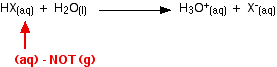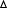Skip to main content

# 1.13 The Bond in a Hydrogen Halide

This page discusses the acidity of the hydrogen halides: hydrogen fluoride, hydrogen chloride, hydrogen bromide and hydrogen iodide. It begins by describing their physical properties and synthesis and then explains what happens when they react with water to make acids such as hydrofluoric acid and hydrochloric acid.

## Physical properties

The hydrogen halides are colorless gases at room temperature, producing steamy fumes in moist air. The boiling points of these compounds are shown in the figure below:Hydrogen fluoride has an abnormally high boiling point for a molecule of its size(293 K or 20°C), and can condense under cool conditions. This is due to the fact that hydrogen fluoride can form hydrogen bonds. Because fluorine is the most electronegative of all the elements, the fluorine-hydrogen bond is highly polarized. The hydrogen atom carries a high partial positive charge (δ+); the fluorine is fairly negatively charged (δ-).

In addition, each fluorine atom has 3 lone pairs of electrons. Fluorine's outer electrons are at the n=2 level, and the lone pairs represent small, highly charged regions of space. Hydrogen bonds form between the δ+ hydrogen on one HF molecule and a lone pair on the fluorine of another one.The figure below illustrates this association:The other hydrogen halides do not form hydrogen bonds because the larger halogens are not as electronegative as fluorine; therefore, the bonds are less polar. In addition, their lone pairs are at higher energy levels, so the halogen does not carry such an intensely concentrated negative charge; therefore, other hydrogen atoms are not attracted as strongly.

### Making hydrogen halides

There are several ways of synthesizing hydrogen halides; the method considered here is the reaction between an ionic halide, like sodium chloride, and an acid like concentrated phosphoric(V) acid, H3PO4, or concentrated sulfuric acid.

### Making hydrogen chloride

When concentrated sulfuric acid is added to sodium chloride under cold conditions, the acid donates a proton to a chloride ion, forming hydrogen chloride. In the gas phase, it immediately escapes from the system.

$Cl^- + H_2SO_4 \rightarrow HCl + HSO_4^-$

The full equation for the reaction is:

$NaCl + H_2SO_4 \rightarrow HCl + NaHSO_4$

Sodium bisulfate is also formed in the reaction. Concentrated phosphoric(V) acid reacts similarly, according to the following equation:

$Cl^- + H_3PO_4 \rightarrow HCl + H_2PO_4^-$

The full ionic equation showing the formation of the salt, sodium biphosphate(V), is given below:

$NaCl + H_3PO_4 \rightarrow HCl + NaH_2PO_4$

### Making other hydrogen halides

All hydrogen halides can be formed by the same method, using concentrated phosphoric(V) acid.

Concentrated sulfuric acid, however, behaves differently. Hydrogen fluoride can be made with sulfuric acid, but hydrogen bromide and hydrogen iodide cannot.

The problem is that concentrated sulfuric acid is an oxidizing agent, and as well as producing hydrogen bromide or hydrogen iodide, some of the halide ions are oxidized to bromine or iodine. Phosphoric acid does not have this ability because it is not an oxidant.

## The acidity of the hydrogen halides

### Hydrogen chloride as an acid

By the Bronsted-Lowry definition of an acid as a proton donor, hydrogen chloride is an acid because it transfers protons to other species. Consider its reaction with water.

Hydrogen chloride gas is soluble in water; its solvated form is hydrochloric acid. Hydrogen chloride fumes in moist air are caused by hydrogen chloride reacting with water vapor in the air to produce a cloud of concentrated hydrochloric acid.

A proton is donated from the hydrogen chloride to one of the lone pairs on a water molecule.A coordinate (dative covalent) bond is formed between the oxygen and the transferred proton.

The equation for the reaction is the following:

$H_2O + HCl \rightarrow H_3O^+ + Cl^-$

The H3O+ ion is the hydroxonium ion (also known as the hydronium ion or the oxonium ion). This is the normal form of protons in water; sometimes it is shortened to the proton form, H+(aq), for brevity.

When hydrogen chloride dissolves in water (to produce hydrochloric acid), almost all the hydrogen chloride molecules react in this way. Hydrochloric acid is therefore a strong acid. An acid is strong if it is fully ionized in solution.

### Hydrobromic acid and hydriodic acid as strong acids

Hydrogen bromide and hydrogen iodide dissolve in (and react with) water in exactly the same way as hydrogen chloride does. Hydrogen bromide forms hydrobromic acid; hydrogen iodide gives hydriodic acid. Both of these are also strong acids.

### Hydrofluoric acid as an exception

By contrast, although hydrogen fluoride dissolves freely in water, hydrofluoric acid is only a weak acid; it is similar in strength to organic acids like methanoic acid. The complicated reason for this is discussed below.

## The bond enthalpy of the H-F bond

Because the fluorine atom is so small, the bond enthalpy (bond energy) of the hydrogen-fluorine bond is very high. The chart below gives values for all the hydrogen-halogen bond enthalpies:

bond enthalpy
(kJ mol-1)
H-F +562
H-Cl +431
H-Br +366
H-I +299

In order for ions to form when the hydrogen fluoride reacts with water, the H-F bond must be broken. It would seem reasonable to say that the relative reluctance of hydrogen fluoride to react with water is due to the large amount of energy needed to break that bond, but this explanation does not hold.

## The energetics of the process from HX(g) to X-(aq)

The energetics of this sequence are of interest:All of these terms are involved in the overall enthalpy change as you convert HX(g) into its ions in water.

However, the terms involving the hydrogen are the same for every hydrogen halide. Only the values for the red terms in the diagram need be considered. The values are tabulated below:

bond enthalpy of HX
(kJ mol-1)
electron affinity of X
(kJ mol-1)
hydration enthalpy of X-
(kJ mol-1)
sum of these
(kJ mol-1)
HF +562 -328 -506 -272
HCl +431 -349 -364 -282
HBr +366 -324 -335 -293
HI +299 -295 -293 -289

There is virtually no difference in the total HF and HCl values.

The large bond enthalpy of the H-F bond is offset by the large hydration enthalpy of the fluoride ion. There is a very strong attraction between the very small fluoride ion and the water molecules. This releases a lot of heat (the hydration enthalpy) when the fluoride ion becomes wrapped in water molecules.

## Other attractions in the system

The energy terms considered previously have concerned HX molecules in the gas phase. To reach a more correct explanation, the molecules must first be considered as unreacted aqueous HX molecules. The equation for this is given below:The equation is incorporated into an improved energy cycle as follows:Unfortunately, values for the first step in the reaction are not readily available. However, in each case, the initial separation of the HX from water molecules is endothermic. Energy is required to break the intermolecular attractions between the HX molecules and water.

That energy is much greater for hydrogen fluoride because it forms hydrogen bonds with water. The other hydrogen halides experience only the weaker van der Waals dispersion forces or dipole-dipole attractions.

The overall enthalpy changes (including all the stages in the energy cycle) for the reactions are given in the table below:

$HX(aq) + H_2O (l) \rightarrow H_3O^+ (aq) + X^- (aq)$

enthalpy change
(kJ mol-1)
HF -13
HCl -59
HBr -63
H-I -57

The enthalpy change for HF is much smaller in magnitude than that for the other three hydrogen halides, but it is still negative exothermic change. Therefore, more information is needed to explain why HF is a weak acid.

## Entropy and free energy considerations

The free energy change, not the enthalpy change, determines the extent and direction of a reaction.

Free energy change is calculated from the enthalpy change, the temperature of the reaction and the entropy change during the reaction.

For simplicity, entropy can be thought of as a measure of the amount of disorder in a system. Entropy is given the symbol S. If a system becomes more disordered, then its entropy increases. If it becomes more ordered, its entropy decreases.

The key equation is given below:In simple terms, for a reaction to happen, the free energy change must be negative. But more accurately, the free energy change can be used to calculate a value for the equilibrium constant for a reaction using the following expression:The term Ka is the equilibrium constant for the reaction below:

$HX(aq) + H_2O(l) \rightleftharpoons H_3O^+(aq) + X^-(aq)$

The values for TΔS (needed to calculate ΔG) for the four reactions at a temperature of 298 K are tabulated below:

TS
(kJ mol-1)
HF -29
HCl -13
HBr -4
H-I +4

Notice that at the top of the group, the systems become more ordered when the HX reacts with the water. The entropy of the system (the amount of disorder) decreases, particularly for the hydrogen fluoride.

The reason for this is that the very strong attraction between H3O+ and F-(aq) imposes a lot of order on the system, as does the attraction between the water molecules and the various ions present. These attractions are each greatest for the small fluoride ions.

The total effect on the free energy change, and therefore the value of the equilibrium constant, can now be considered. These values are calculated in the following table:H
(kJ mol-1)
TS
(kJ mol-1)G
(kJ mol-1)
Ka
(mol dm-3)
HF -13 -29 +16 1.6 x 10-3
HCl -59 -13 -46 1.2 x 108
HBr -63 -4 -59 2.2 x 1010
HI -57 +4 -61 5.0 x 1010

The values for these estimated equilibrium constants for HCl, HBr and HI are so high that the reaction can be considered "one-way". The ionization is virtually 100% complete. These are all strong acids, increasing in strength down the group.

By contrast, the estimated Ka for hydrofluoric acid is small. Hydrofluoric acid only ionizes to a limited extent in water. Therefore, it is a weak acid.

The estimated value for HF in the table can be compared to the experimental value:

• Experimental value: 5.6 x 10-4 mol dm-3
• Estimated value: 1.6 x 10-3 mol dm-3

These values differ by an order of magnitude, but because of the logarithmic relationship between the free energy and the equilibrium constant, a very small change in ΔG has a very large effect on Ka.

To have the values in close agreement, ΔG would must increase from +16 to +18.5 kJ mol-1. Given the uncertainty in the values used to calculate ΔG, the difference between the calculated value and the experimental value could easily fall within this range.

## Summary: Why is hydrofluoric acid a weak acid?

The two main factors are:

• Entropy decreases dramatically when the hydrogen fluoride reacts with water. This is particularly noticeable with hydrogen fluoride because the attraction of the small fluoride ions produced imposes significant order on the surrounding water molecules and nearby hydronium ions. The effect decreases with larger halide ions.
• Very strong hydrogen bonding exists between the hydrogen fluoride molecules and water molecules. This costs a large amount of energy to break. This effect does not occur in the other hydrogen halides.

## Contributors

Jim Clark (Chemguide.co.uk)